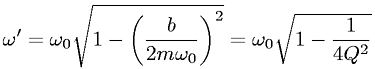Equations > Physics > Oscillations and Waves > Angular frequency for a damped oscillation

### Angular frequency for a damped oscillationLatex Code:

MathML Code:

 $\omega \text{'}={\omega }_{0}\sqrt{1-{\left(\fracb2m{\omega }_{0}\right)}^{2}}={\omega }_{0}\sqrt{1-\frac14{Q}^{2}}$

MathType 5.0: